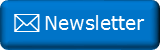﻿ Nine digits puzzleNewsletter: Click here to join an email list and be contacted when a new article is published.      Advertisement

# Nine digits Puzzle

Here's a little coffee-time challenge puzzle

ABCDEFGHI

Arrange the digits 1-9 to make a nine digit number such that:

• The first digit, A is divisible by one.

• The first two digits, AB is divisible by two.

• The first three digits, ABC is divisible by three.

• The first four digits, ABCD is divisible by four.

• The first five digits, ABCDE is divisible by five …

• … All nine digits, ABCDEFGHI is divisible by nine.When I first saw this puzzle, I immediately thought of a brute force approach; a few lines of code and you can permute through every possible combination of the digits in a few seconds, but by applying a couple of the simple divisibility rules we're taught in schools, you can solve this with pen, paper, and a half a cup of coffee. If you need a refresher on simple divisibility tests, you can find one here.

Give it a go, then check your solution below:

You can find a complete list of all the articles here.Click here to receive email alerts on new articles.